# NCERT Solutions For Class 6 Maths Basic Geometrical Ideas Exercise 4.6

NCERT Solutions For Class 6 Maths Basic Geometrical Ideas Exercise 4.6

### NCERT Solutions For Class 6 Maths Basic Geometrical Ideas Exercise 4.6

NCERT Solutions for Class 6 Maths Chapter 4 Basic Geometrical Ideas Ex 4.6

Exercise 4.6

Ex 4.6 Class 6 Maths Question 1.
From the figure, identify:
(a) the centre of circle
(c) a diameter
(d) a chord
(e) two points in the interior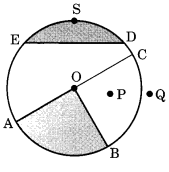(f) a point in the exterior
(g) a sector
(h) a segment.
Solution:
In the given figure,Ex 4.6 Class 6 Maths Question 2.
(a) Is every diameter of a circle also a chord?
(b) Is every chord of a circle also a diameter?
Solution:
(a) Yes, every diameter is the longest chord of a circle.
(b) No, every chord is not diameter of a circle.

Ex 4.6 Class 6 Maths Question 3.
Draw any circle and mark
(a) its centre
(c) a diameter
(d) a sector
(e) a segment
(f) a point in its interior
(g) a point in its exterior
(h) an arc.
Solution:
In the given circle,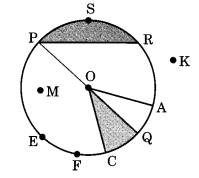Ex 4.6 Class 6 Maths Question 4.
Say ‘true’ or ‘false’.
(a) Two diameters of a circle will necessarily intersect.
(b) The centre of a circle is always in its interior.
Solution:
(a) True
(b) True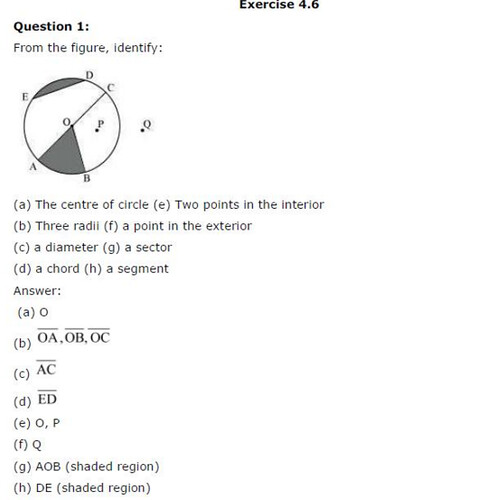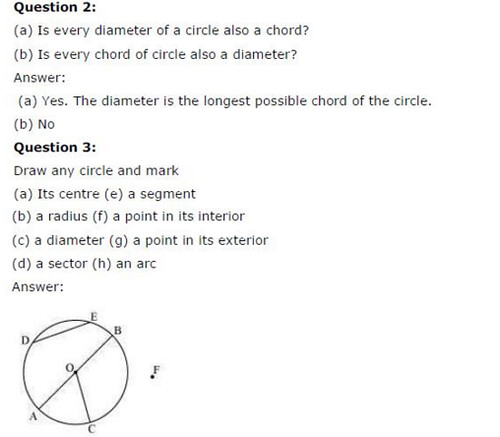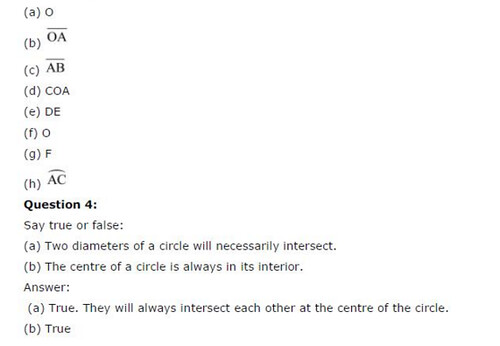## SabDekho

The Complete Educational Website